## //Analog clock script- By Kurt (kurt.grigg@virgin.net) //Script featured on Dynamic Drive //Visit http://www.dynamicdrive.com for this script and more fCol='444444'; //face colour. sCol='FF0000'; //seconds colour. mCol='444444'; //minutes colour. hCol='444444'; //hours colour. Ybase=30; //Clock height. Xbase=30; //Clock width. H='...'; H=H.split(''); M='....'; M=M.split(''); S='.....'; S=S.split(''); NS4=(document.layers); NS6=(document.getElementById&&!document.all); IE4=(document.all); Ypos=0; Xpos=0; dots=12; Split=360/dots; if (NS6){ for (i=1; i < dots+1; i++){ document.write('<div id="n6Digits'+i+'" style="position:absolute;top:0px;left:0px;width:30px;height:30px;font-family:Arial;font-size:10px;color:#'+fCol+';text-align:center;padding-top:10px">'+i+'</div>'); } for (i=0; i < M.length; i++){ document.write('<div id="Ny'+i+'" style="position:absolute;top:0px;left:0px;width:2px;height:2px;font-size:2px;background:#'+mCol+'"></div>'); } for (i=0; i < H.length; i++){ document.write('<div id="Nz'+i+'" style="position:absolute;top:0px;left:0px;width:2px;height:2px;font-size:2px;background:#'+hCol+'"></div>'); } for (i=0; i < S.length; i++){ document.write('<div id="Nx'+i+'" style="position:absolute;top:0px;left:0px;width:2px;height:2px;font-size:2px;background:#'+sCol+'"></div>'); } } if (NS4){ dgts='1 2 3 4 5 6 7 8 9 10 11 12'; dgts=dgts.split(' ') for (i=0; i < dots; i++){ document.write('<layer name=nsDigits'+i+' top=0 left=0 height=30 width=30><center><font face=Arial size=1 color='+fCol+'>'+dgts[i]+'</font></center></layer>'); } for (i=0; i < M.length; i++){ document.write('<layer name=ny'+i+' top=0 left=0 bgcolor='+mCol+' clip="0,0,2,2"></layer>'); } for (i=0; i < H.length; i++){ document.write('<layer name=nz'+i+' top=0 left=0 bgcolor='+hCol+' clip="0,0,2,2"></layer>'); } for (i=0; i < S.length; i++){ document.write('<layer name=nx'+i+' top=0 left=0 bgcolor='+sCol+' clip="0,0,2,2"></layer>'); } } if (IE4){ document.write('<div style="position:absolute;top:0px;left:0px"><div style="position:relative">'); for (i=1; i < dots+1; i++){ document.write('<div id="ieDigits" style="position:absolute;top:0px;left:0px;width:30px;height:30px;font-family:Arial;font-size:10px;color:'+fCol+';text-align:center;padding-top:10px">'+i+'</div>'); } document.write('</div></div>') document.write('<div style="position:absolute;top:0px;left:0px"><div style="position:relative">'); for (i=0; i < M.length; i++){ document.write('<div id=y style="position:absolute;width:2px;height:2px;font-size:2px;background:'+mCol+'"></div>'); } document.write('</div></div>') document.write('<div style="position:absolute;top:0px;left:0px"><div style="position:relative">'); for (i=0; i < H.length; i++){ document.write('<div id=z style="position:absolute;width:2px;height:2px;font-size:2px;background:'+hCol+'"></div>'); } document.write('</div></div>') document.write('<div style="position:absolute;top:0px;left:0px"><div style="position:relative">'); for (i=0; i < S.length; i++){ document.write('<div id=x style="position:absolute;width:2px;height:2px;font-size:2px;background:'+sCol+'"></div>'); } document.write('</div></div>') } function clock(){ time = new Date (); secs = time.getSeconds(); sec = -1.57 + Math.PI * secs/30; mins = time.getMinutes(); min = -1.57 + Math.PI * mins/30; hr = time.getHours(); hrs = -1.57 + Math.PI * hr/6 + Math.PI*parseInt(time.getMinutes())/360; if (NS6){ Ypos=window.pageYOffset+window.innerHeight-Ybase-25; Xpos=window.pageXOffset+window.innerWidth-Xbase-30; for (i=1; i < dots+1; i++){ document.getElementById("n6Digits"+i).style.top=Ypos-15+Ybase*Math.sin(-1.56 +i *Split*Math.PI/180) document.getElementById("n6Digits"+i).style.left=Xpos-15+Xbase*Math.cos(-1.56 +i*Split*Math.PI/180) } for (i=0; i < S.length; i++){ document.getElementById("Nx"+i).style.top=Ypos+i*Ybase/4.1*Math.sin(sec); document.getElementById("Nx"+i).style.left=Xpos+i*Xbase/4.1*Math.cos(sec); } for (i=0; i < M.length; i++){ document.getElementById("Ny"+i).style.top=Ypos+i*Ybase/4.1*Math.sin(min); document.getElementById("Ny"+i).style.left=Xpos+i*Xbase/4.1*Math.cos(min); } for (i=0; i < H.length; i++){ document.getElementById("Nz"+i).style.top=Ypos+i*Ybase/4.1*Math.sin(hrs); document.getElementById("Nz"+i).style.left=Xpos+i*Xbase/4.1*Math.cos(hrs); } } if (NS4){ Ypos=window.pageYOffset+window.innerHeight-Ybase-20; Xpos=window.pageXOffset+window.innerWidth-Xbase-30; for (i=0; i < dots; ++i){ document.layers["nsDigits"+i].top=Ypos-5+Ybase*Math.sin(-1.045 +i*Split*Math.PI/180) document.layers["nsDigits"+i].left=Xpos-15+Xbase*Math.cos(-1.045 +i*Split*Math.PI/180) } for (i=0; i < S.length; i++){ document.layers["nx"+i].top=Ypos+i*Ybase/4.1*Math.sin(sec); document.layers["nx"+i].left=Xpos+i*Xbase/4.1*Math.cos(sec); } for (i=0; i < M.length; i++){ document.layers["ny"+i].top=Ypos+i*Ybase/4.1*Math.sin(min); document.layers["ny"+i].left=Xpos+i*Xbase/4.1*Math.cos(min); } for (i=0; i < H.length; i++){ document.layers["nz"+i].top=Ypos+i*Ybase/4.1*Math.sin(hrs); document.layers["nz"+i].left=Xpos+i*Xbase/4.1*Math.cos(hrs); } } if (IE4){ Ypos=document.body.scrollTop+window.document.body.clientHeight-Ybase-20; Xpos=document.body.scrollLeft+window.document.body.clientWidth-Xbase-20; for (i=0; i < dots; ++i){ ieDigits[i].style.pixelTop=Ypos-15+Ybase*Math.sin(-1.045 +i *Split*Math.PI/180) ieDigits[i].style.pixelLeft=Xpos-15+Xbase*Math.cos(-1.045 +i *Split*Math.PI/180) } for (i=0; i < S.length; i++){ x[i].style.pixelTop =Ypos+i*Ybase/4.1*Math.sin(sec); x[i].style.pixelLeft=Xpos+i*Xbase/4.1*Math.cos(sec); } for (i=0; i < M.length; i++){ y[i].style.pixelTop =Ypos+i*Ybase/4.1*Math.sin(min); y[i].style.pixelLeft=Xpos+i*Xbase/4.1*Math.cos(min); } for (i=0; i < H.length; i++){ z[i].style.pixelTop =Ypos+i*Ybase/4.1*Math.sin(hrs); z[i].style.pixelLeft=Xpos+i*Xbase/4.1*Math.cos(hrs); } } setTimeout('clock()',100); } clock(); //--> Janie and Toni's Viking River Cruise

Posting # 17 on October 23, 2014 ..... Back to the welcome page and listings for other links

Party on the ship! Staff put on a fabulous German bash with oompah music, schnapps, wine beer & headaches for everyone this morning--well not everyone---heh heh

Our email address is janie8500@gmail.com. Please drop a note and say hello anytime!

 1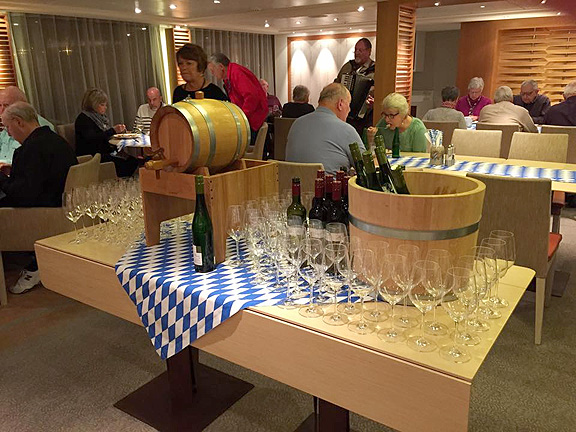2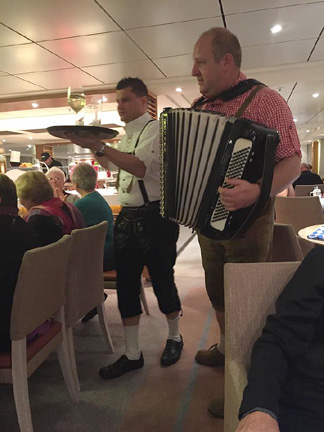34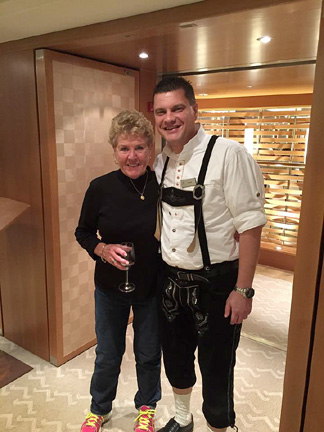5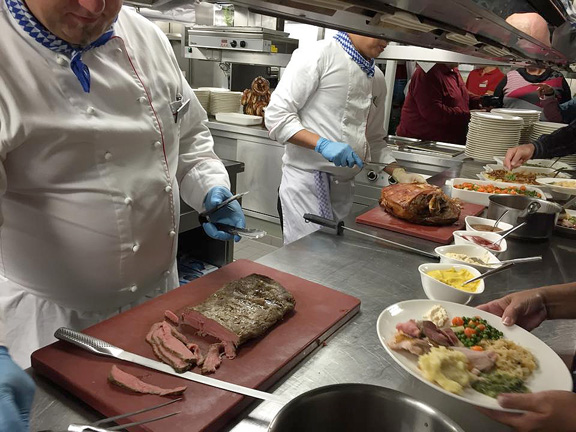6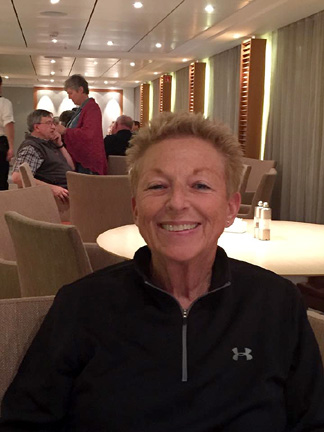Back to the welcome page and listings for other linksAny mistakes or corrections, email me at coachellis@cox.net